Breaking News
Home / Computer / √ 10+ Examples of Simple Flowcharts & Algorithms [+PENJELASAN]# √ 10+ Examples of Simple Flowcharts & Algorithms [+PENJELASAN]

Did you know that at the beginning of making an application, both in mobile, web and desktop must first be designed in algorithms and flowcharts. A programmer will not know the process of coding if he does not know what the program is like.

Therefore, if you want to be a developer or programmer, you must learn about algorithms and flowcharts so you know the application what you make for what purpose.

## Examples of Flowcharts with Analysis and Algorithms

To find out more about the use of from the flowchart you can see examples of flowcharts and their analysis and algorithms below:  1. Flowchart for Calculating Circumference of Circles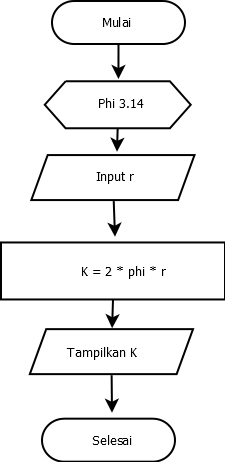Analysis of calculating the circumference of a circle, namely:

• Input: r (circle radius) and phi
• Circumference Circle K = 2 * phi * r

The algorithm of calculating the circumference of a circle, namely:

• First, you must specify the value of phi, as we have seen that the value of phi is 3.14
• Next you have to count the radius of the circle  Then you can enter the formula to calculate the circumference of the circle, which is the formula K = 2 * phi * r
• After entering the formula, the K value will be displayed or printed as an output on the output device.  2. Flowcharts for Sending Letters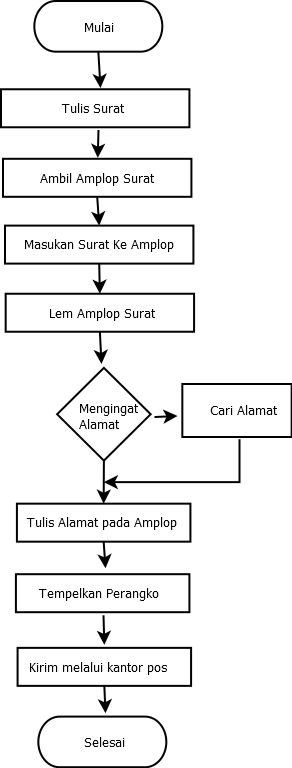Algorithms of sending letters, namely:

• First thing to do is typing or writing
• Then have to prepare an envelope or letter cover
• Then insert the letter into an envelope that has been provided previously
• After inserting the letter into an envelope, you must attach the envelope to the letter using glue
• Next, it must write down the address of the sending letter, if you do not remember, so it is better to take the address book and also look for the address that will be addressed beforehand. Then write the address on the letter envelope.
• After that, buy a postage stamp and paste the stamp on the envelope.
• The final step is that you have to go to the post office and bring the letter to the postal employee.

### 3. Flowchart for Determining Even and Odd NumbersAlgorithms of determining an number are even or odd, namely:

• First is inputting or entering a number, the number must be an integer
• Then divide the entered number by 2
• If the result of the remainder of the division is 0, it can be concluded that the number is even
• Conversely, if the result of the remainder is not 0, it can be concluded that the number is odd

### 4. Flowchart for Temperature Conversion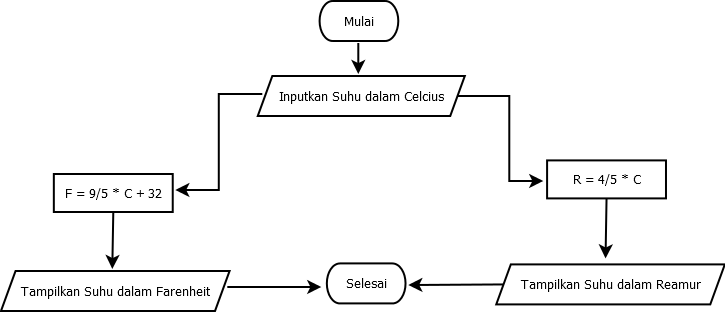Algorithms of converting temperatures, namely:

• First is inputting or entering the temperature amount into Celsius
• If a temperature is to change from Celsius to Reamur then you have to use the formula R = 4/5 * C
• And if a temperature is to be changed from Celsius to Fahrenheit then you have to use the formula F = 9/5 * C + 3
• After that the temperature at the Fahrenheit or Reamur amount will be displayed

### 5. Flowchart for Making a Cup of CoffeeAlgorithm of making a cup of coffee, namely:

• The first thing to do is you have to open the sachet coffee that was purchased
• Then pour the coffee in a glass
• Then you can heat the water to boiling
• And then you can pour the hot water into a glass
• Then you have to stir the coffee evenly
• And coffee also ready to drink

### 6. Food Ordering Flowchart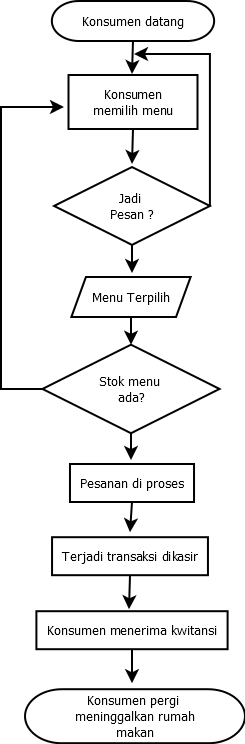Algorithm of ordering food, namely:

• The first phase is the customer will stop by the restaurant
• Then the customer will choose the food choice on the menu provided
• If the food is according to the tastes of the customer, then the customer will order food. Whereas if the food on the menu does not suit the tastes of the customer, they will not place an order
• If the customer is to eat food then the menu will be selected
• A waiter will later see the existing food stock, if available, the food will be processed. Whereas if it is not available, the waiter will advise the customer to choose another menu
• Then after it is processed, the customer will receive the food he has ordered
• After that a payment transaction will occur at the counter
• and a guard the cashier will then provide proof of payment or receipt
• If all these stages have been passed, then the customer will leave the restaurant

### 7. Flowchart for Calculating Final Value Results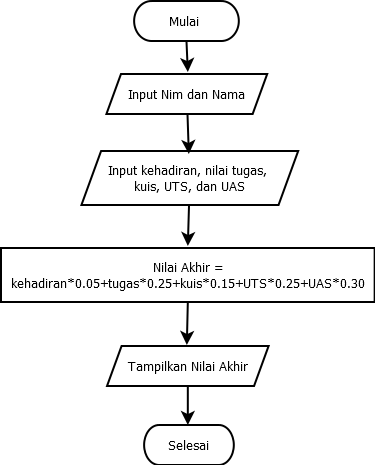Algorithms of Calculating Final Value Results, namely:

• First time inputting or entering Nim and Student Name
• Then input or enter Attendance (Attendance), assignments, quizzes, Semester Semester Exams and Semester Final Examinations
• Next, enter the formula of the final score, namely: attendance * 0.05 + assignments * 0.25 + quiz * 0.15 + UTS * 0.25 + UAS * 0.30
• Lastly, only the final result is displayed

### 8. Flowchart for Counting Hours into Secondary Units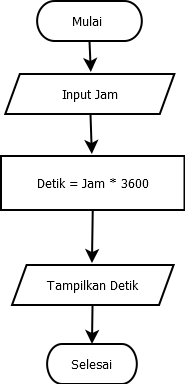Algorithms of Counting hours in seconds, namely:

• First is inputting or entering the Clock
• Next is calculating using a formula, namely Hours * 3600
• Finally, it displays results that have changed to seconds

### 9. Flowchart for Calculating KM into a Meter UnitAlgorithms of Calculating KM in meters, namely:

• First, input or enter KM values ​​
• , namely KM * 1000
• Lastly, it displays results that have changed to meters

### 10. Flowchart for Calculating the Area of ​​a RectangleAnalysis of calculating the area of ​​a rectangle, namely:

• Input: p (length) and l (width)
• L = p * l

Algorithm of Calculating the area of ​​a rectangle, namely:

• First to do is input or enter a long value
• Second, input or enter the value width
• calculate L, namely L = p * l
• Finally the L value will be displayed as an output

That is example flowchart along with the analysis and algorithm you need to know. Hopefully useful and can increase your knowledge about flowcharts.

Summary

Here is a collection of examples of flowcharts and their analysis and algorithms that are easy to understand by beginners.